# How to Subtract Fractions with Variables

Instructor: Laura Pennington

Laura received her Master's degree in Pure Mathematics from Michigan State University. She has 15 years of experience teaching collegiate mathematics at various institutions.

In this lesson, we will learn the steps involved with subtracting fractions with variables. After discussing the steps, we will look at an example of how to apply these steps to really solidify our understanding of the process.

## Steps to Solve

When it comes to subtracting fractions with variables, we take the problem through the same steps as if we were subtracting fractions without variables. However, because of the variables, the simplification process is different. When we are subtracting fractions with variables, the first thing we want to do is get a common denominator. To find the common denominator we simply multiply the two denominators together.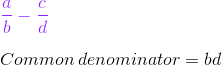The second step is to manipulate each fraction so that they each have this common denominator. To do this, we use the rule that anything we multiply the denominator by, we must also multiply the numerator by.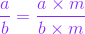The first and second step can be carried out using the following formula.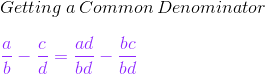We have that (a / b) - (c / d) = (ad / bd) - (bc / bd), where a, b, c, and d can be numbers, variables, or expressions.

Are you with me so far? I know that sometimes imagining things in the general sense can be hard! Don't worry, though. In the last section of this lesson, we will look at an example of how to apply these steps so that we can become really comfortable with the process.

Alright, back to these steps. We now have a common denominator. The third step in the process is to subtract the numerators. We can add this step on to our formula above to get (a / b) - (c / d) = (ad / bd) - (bc / bd) = (ad - bc) / bd.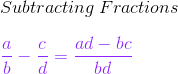The fourth step is where the simplification comes in. Once we've performed our subtraction, we need to simplify the resulting fraction. We do this by factoring the numerator and denominator as much as possible and then cancelling out any common factors that are in both. Once you've completed step four, you're all set!

## Solution

The steps we follow to subtract fractions with variables are as follows.

1. Find a common denominator by multiplying the two denominators together.
2. Manipulate the fractions so that they both have the common denominator.
3. Once you have a common denominator in both fractions, subtract the numerators.
4. Simplify the resulting fraction from step three by factoring the numerator and denominator as much as possible and cancelling out any common factors that are in both.

The formula we use for steps 1-3 is as follows.## Applying the Steps

Okay, now that we've got a general idea of what we need to do to subtract fractions with variables, let's take a look at applying these steps to an actual problem. This will really help to solidify our understanding!

Suppose you are working on a problem with two unknowns, and you get to a point in the problem where you need to subtract (3x / 6x 2) - (2y / 4y 3).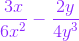This is a case where we are subtracting fractions with variables. Great! We can practice using our steps!

The first step is to find a common denominator by multiplying the two denominators together. This gives 6x 2 * 4y 3 = 24x 2y 3. That was easy enough!

The second step is to manipulate the fractions to get that common denominator on both of them. We can use our formula to do this.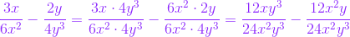To unlock this lesson you must be a Study.com Member.

### Register to view this lesson

Are you a student or a teacher?

#### See for yourself why 30 million people use Study.com

##### Become a Study.com member and start learning now.
Back
What teachers are saying about Study.com

### Earning College Credit

Did you know… We have over 200 college courses that prepare you to earn credit by exam that is accepted by over 1,500 colleges and universities. You can test out of the first two years of college and save thousands off your degree. Anyone can earn credit-by-exam regardless of age or education level.